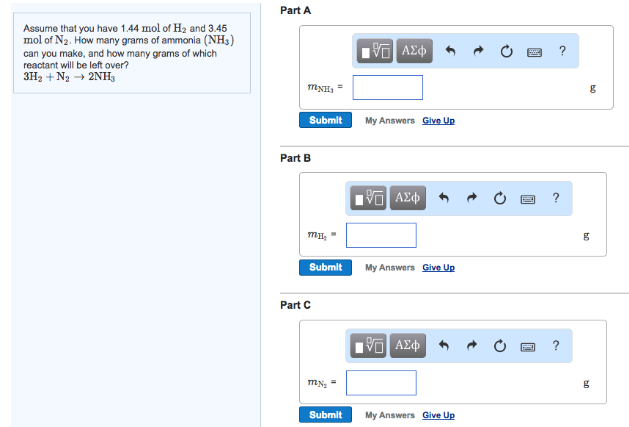# Problem: Assume that you have 1.44 mol of H2 and 3.45 mol of N2. How many grams of ammonia (NH3) can you make, and how many grams of which reactant will be left over? 3H2 + N2 → 2NH3mNH3 = mH2 = mN2  =

###### FREE Expert Solution
100% (255 ratings)###### Problem Details

Assume that you have 1.44 mol of H2 and 3.45 mol of N2. How many grams of ammonia (NH3) can you make, and how many grams of which reactant will be left over?

3H2 + N2 → 2NH3

mNH

mH2

mN =